## How to Calculate and Solve for the Volume and Radius of a Sphere | The Calculator Encyclopedia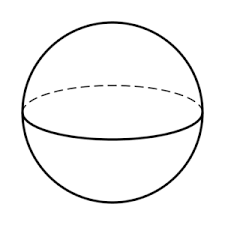The image above is a sphere.

To compute the volume of a sphere, one essential parameter is needed and this parameter is the radius of the sphere (r). You can also use diameter of the sphere (d).

The formula for calculating the volume of a sphere:

V = (4/3)πr³

Where;

V = Volume of the sphere
r = Radius of the sphere

Let’s solve an example:
Find the volume of a sphere when the radius of the sphere is 10 cm.

This implies that;

r = Radius of the sphere = 10 cm

V = (4/3)πr³
V = (4/3)π x (10)³
V = (4/3)π x 1000
V = (4/3)3.142 x 1000
V = (4/3)3142
V = 1.33 x 3142
V = 4188.7

Therefore, the volume of the sphere is 4188.7 cm³.

Calculating the Volume of a Sphere using Diameter of the Sphere.

V = 4πd³ / 24

Where;

V = Volume of the sphere
d = Diameter of the sphere

Let’s solve an example:
Find the volume of a sphere when the diameter of the sphere is 20 cm.

This implies that;

d = Diameter of the sphere = 20 cm

V = 4πd³ / 24
V = 4 x 3.142 x (20)³ / 24
V = 4 x 3.142 x 8000 / 24
V = 100544 / 24
V = 4189.3

Therefore, the volume of the sphere with diameter is 4189.3 cm3.

Calculating the Radius of a Sphere using Volume of the Sphere.

r = 3√(3V / )

Where;

V = Volume of the sphere
r = Radius of the sphere

Let’s solve an example:
Find the radius of a sphere when the volume of the sphere is 250 cm3.

This implies that;

V = Volume of the sphere = 250 cm3

r = 3√(3V / )
r = 3√(3 x 250 / 4 x 3.142)
r = 3√(750 / 12.568)
r = 3√59.675
r = 3.907

Therefore, the radius of the sphere is 3.907 cm.

Calculating the Diameter of a Sphere using Volume of the Sphere.

d = 3√(24V / )

Where;

A = Area of the sphere
d = Diameter of the sphere

Let’s solve an example:
Find the diameter of a sphere when the volume of the sphere is 40 cm3.

This implies that;

V = Volume of the sphere = 40 cm3

d = 3√(24V / )
d = 3√(24 x 40 / 4 x 3.142)
d = 3√(960 / 12.568)
d = 3√76.38
d = 4.24

Therefore, the diameter of the sphere is 4.24 cm.

## How to Calculate and Solve for the Area and Radius of a Sphere | Nickzom Calculator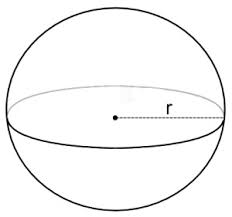The image above is a sphere.

To compute the area of a sphere, one essential parameter is needed and this parameter is the radius of the sphere (r). You can also use diameter of the sphere (d).

The formula for calculating the area of a sphere:

A = 4πr²

Where;

A = Area of the sphere
r = Radius of the sphere

Let’s solve an example:
Find the area of a sphere when the radius of the sphere is 6 cm.

This implies that;

r = Radius of the sphere = 6 cm

A = 4πr²
A = 4 x 3.142 x 6²
A = 4 x 3.142 x 36
A = 452.4

Therefore, the area of the sphere is 452.4 cm2.

Calculating the Area of a sphere using Diameter of the sphere.

A = πd2

Where;

A = Area of the sphere
d = Diameter of the sphere

Let’s solve an example:
Find the area of a sphere when the diameter of the sphere is 8 cm.

This implies that;

d = Diameter of the sphere = 8 cm

A = πd2
A = 3.142 x 82
A = 3.142 x 64
A = 201.08

Therefore, the area of the sphere is 201.08 cm2.

Calculating the Radius of a sphere using Area of the sphere.

r = √(A / )

Where;

A = Area of the sphere
r = Radius of the sphere

Let’s solve an example:
Find the radius of a sphere when the area of the sphere is 22 cm2.

This implies that;

A = Area of the sphere = 22 cm2

r = √(A / )
r = √(22 / 4 x 3.142)
r = √(22 / 12.57)
r = √1.75
r = 1.32

Therefore, the radius of the sphere is 1.32 cm.

## How to Calculate and Solve for the Area, Radius, Diameter and Slant Height of a Cone | The Calculator Encyclopedia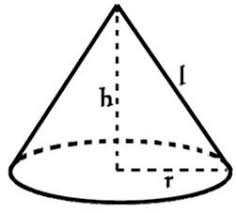The image above is a cone.

To compute the area of a cone, two essential parameters is needed and this parameters are the radius of the cone (r) and the slant height of the cone (h).

The formula for calculating the area of a cone:

A = πrl + πr²

Where;

A = Area of the Cone
r = Radius of the Cone

Let’s solve an example:
Find the area of a cone when the radius of the cone is 9 cm and the slant height of the cone is 12 cm.

This implies that;
r = Radius of the cone = 9 cm
l = Slant height of the cone = 12 cm

A = πrl + πr²
A = 3.142 x 9 x 12 + 3.142 x 9²
A = 339.336 + 254.502
A =  593.83

Therefore, the area of the cone is 593.83 cm².

Calculating the Area of a cone using Diameter and Slant height of the cone.

A = πdl / 2 + πd2 / 4

Where;

d = Diameter of the Cone
l = Slant height of the Cone

Let’s solve an example:
Find the area of a cone when the diameter of the cone is 18 cm and the slant height of the cone is 22 cm?

This implies that;
d = diameter of the cone = 18 cm
l = Slant height of the cone = 22 cm

A = πdl / 2 + πd2 / 4
A = 3.142 x 18 x 22 / 2 + 3.142 (18)2 / 4
A = 1244.232 / 2 + 1018.008 / 4
A = 622.116 + 254.502
A = 876.6

Therefore, the area of the cone with diameter is 876.6 cm2.

Calculating the Slant height of a cone using Radius of the cone and Area of the cone.

l = A – πr2 / πr

Where;

A = Area of the Cone
r = Radius of the Cone

Let’s solve an example:
Find the slant height of a cone when the radius of the cone is 8 cm and the area of the cone is 220 cm2.

This implies that;
A = Area of the cone = 220 cm2
r = Radius of the cone = 8 cm

l = A – πr2 / πr
l = 220 – 3.142 x 82 / 3.142 x 8
l = 220 – 3.142 x 64 / 25.136
l = 220 – 201.088 / 25.136
l = 18.91 / 25.136
l = 0.75

Therefore, the slant height of the cone with radius is 0.75 cm.

## How to Calculate and Solve for the Perimeter or Circumference, Diameter, Radius and Angle of a Sector | The Calculator Encyclopedia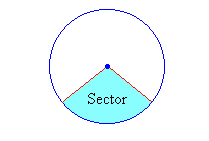The image above is a sector.

To compute the Perimeter or Circumference a sector, two essential parameters is needed and this parameters are the radius of the sector (r) and the angle of the sector (θ). You can also use the diameter of the sector (d).

The formula for calculating the Perimeter or Circumference of a sector:

P = 2r + θ / 360(2πr)

Where;

P = Perimeter or Circumference
r = Radius of the sector
θ = Angle of the Sector

Let’s solve an example:
Find the perimeter or circumference of a sector when the radius of the sector is 14 cm and the angle of the sector is 60°

This implies that;

r = Radius of the sector = 14 cm
θ = Angle of the sector = 60°

P = 2r + θ / 360(2πr)
P = 2 x 14 + 60 / 360(2 x 3.142 x 14)
P = 28 + 0.1667 (87.97)
P = 28 + 14.66
P = 42.66

Therefore, the perimeter or circumference of the sector is 42.66 cm.

Calculating the Perimeter or Circumference of a Sector using Diameter and Angle of the sector.

P = d + θ / 360(πd)

θ = Angle of the sector
d = Diameter of the sector

Let’s solve an example;
Find the perimeter or circumference of a sector when the diameter of the sector is 20 cm and the angle of the sector is 80°.

This implies that;

d = Diameter of the sector = 20 cm
θ = Angle of the sector = 80°

## How to Calculate and Solve for the Area, Radius, Diameter and Angle of a Sector | The Calculator Encyclopedia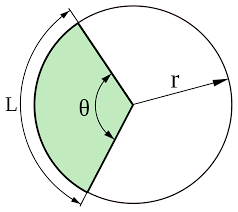The image above is a sector.

To compute the area of a sector, two essential parameters is needed and this parameters are the radius of the sector (r) and the angle of the sector (θ). You can also use the diameter of the sector (d).

The formula for calculating the area of a sector:

Area of a sector = (θ/360)[πr²]

Where;

A = Area of the Sector
r = Radius of the Sector
θ = Angle of the Sector

Let’s solve an example:
Find the area of a sector when the radius of the sector is 7 cm and the angle of the sector is 9°

This implies that;

r = Radius of the sector = 7 cm
θ = Angle of the sector = 9°

A = θ / 360 x πr2
A = (9/360)[π x 7²]
A = 0.025 x π x 49
A = 3.848

Therefore, the area of the sector is 3.848 cm2.

Calculating the Area of a Sector using Diameter and Angle of the sector.

The formula is A = θ / 360 x πd2 / 4

Where;

θ = Angle of the sector
d = Diameter of the sector

Let’s solve an example;
Find the Area of a sector when the diameter of the sector is 12 cm and the angle of the sector is 18°.

This implies that;

d = Diameter of the sector = 12 cm
θ = Angle of the sector = 18°

A = θ / 360 x πd2 / 4
A = 18 / 360 x 3.142 (144) / 4
A = 18 / 360 x 452.448 / 4
A= 18 / 360 x 113.112
A= 0.05 x 113.112
A = 5.656

Therefore, the area of the sector with diameter is 5.656 cm

How to Calculate Angle of a Sector when Area of the Sector and Radius of the Sector is Given

θ = 360 (A) / πr2

where;

r = Radius of a sector
A = Area of a sector

Let’s solve an example;
Given that the area of a sector is 15 cm2 and the radius of the sector is 5 cm. Find the angle of the sector?

This implies that;
A = Area of the sector = 15 cm2
r = Radius of the sector = 5 cm

θ = 360 (A) / πr2
θ = 360 (15) / 3.142 (5)2
θ = 5400 / 3.142 (25)
θ = 5400 / 78.55
θ = 68.746

Therefore, the angle of the sector is 68.746°.

How to Calculate Angle of a Sector when Area of the Sector and Diameter of the Sector is Given

θ = 1440 (A) / πd2

where;

d = Diameter of a sector
A = Area of a sector

Let’s solve an example;
Given that the area of a sector is 22 cm2 and the diameter of the sector is 10 cm. Find the angle of the sector?

This implies that;
A = Area of the sector = 22 cm2
d = Diameter of the sector = 10 cm

θ = 1440 (A) / πr2
θ = 1440 (22) / 3.142 (10)2
θ = 31680 / 3.142 (100)
θ = 31680 / 314.2
θ = 100.88

Therefore, the angle of the sector is 100.88°.

How to Calculate Diameter of a Sector when Area of the Sector and Angle of the Sector is Given

d = √1440 (A) / πθ

where;

θ = Angle of a sector
A = Area of a sector

Let’s solve an example;
Given that the area of a sector is 24 cm2 and the angle of the sector is 10°. Find the diameter of the sector?

This implies that;
A = Area of the sector = 24 cm2
θ = Angle of the sector = 10°

d = √1440 (A) / πθ
d = √1440 (24) / 3.142 x 10
d = √34560 / 31.42
d = √1099.936
d = 33.165

Therefore, the diameter of the sector is 33.165 cm.

How to Calculate Radius of a Sector when Area of the Sector and Angle of the Sector is Given

r = √360 (A) / πθ

where;

θ = Angle of a sector
A = Area of a sector

## How to Calculate and Solve for the Volume, Radius and Height of a Cylinder | Nickzom Calculator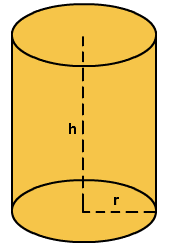The image above represents a cylinder.
To compute the volume of a cylinder requires two essential parameters which are the radius and height of the cylinder.

The formula for computing the volume of a cylinder is:

V = πr2h

Where:
V = Volume of a cylinder
r = radius of the cylinder
h = height of the cylinder

Let’s solve an example
Find the volume of a cylinder with a radius of 3 cm and a height of 5 cm.

This implies that:
r = radius of the cylinder = 3
h = height of the cylinder = 5

V = πr2h
V = 3.142 x 32 x 5
V = 141.39

Therefore, the volume of the cylinder is 141.39 cm3.

Calculating the Height of a cylinder when Volume and Radius is Given

The formula is h = V / πr2

Where;
V = Volume of a cylinder
r =  radius of the cylinder
h = height of the cylinder

Let’s solve an example:
Find the height of a cylinder with a volume of 300 cm3 and a radius of 3 cm

This implies that;
V = Volume of the cylinder = 300 cm3
r  = radius of the cylinder = 3 cm

h = V / πr2
h = 300 / 3.142(3)2
h = 300 / 28.278
h = 10.61
Therefore, the height of the cylinder is 10.61 cm.

Calculating the Radius of a cylinder when Volume and Height is Given

The formula is r = √(V / πh)

Where;
V = Volume of a cylinder
r =  radius of the cylinder
h = height of the cylinder

Let’s solve an example:
Find the radius of a cylinder with a volume of 200 cm3 and a height of 5 cm

This implies that;
V = Volume of the cylinder = 200 cm3
h  = height of the cylinder = 5 cm

r = √(V / πh)
r = √(200 / 3.142(5))
r = √(200 / 15.71)
r = √12.73
r = 3.57

Therefore, the radius of the cylinder is 3.57 cm.

## How to Calculate the Area, Radius and Height of a Cylinder | Nickzom Calculator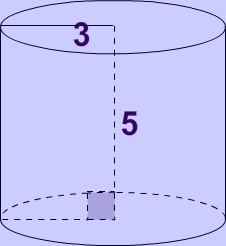The image above represents a cylinder.
To compute the area of a cylinder requires two essential parameters which are the radius and height of the cylinder.

The formula for computing the area of a cylinder is:

A = 2πrh + 2πr2

Where:
A = Area of a cylinder
r = radius of the cylinder
h = height of the cylinder

Let’s solve an example
Find the area of a cylinder with a radius of 3 cm and a height of 5 cm.

This implies that:
r = radius of the cylinder = 3
h = height of the cylinder = 5

A = 2πrh + 2πr2
A = 2(3.142) x 3 x 5 + 2(3.142) x 32
A = 150.82

Therefore, the area of the cylinder is 150.82 cm2.

Calculating the Height of a cylinder when Area and Radius is Given

The formula is h =  A/ 2πr – r

Where;
A = Area of a cylinder
r =  radius of the cylinder
h = height of the cylinder

Let’s solve an example:
Find the height of a cylinder with an area of 600 cm² and a radius of 5 cm

This implies that;
A = Area of the cylinder = 600 cm²
r  = radius of the cylinder = 5 cm

h = A / 2πr – r
h = 600 / 2(3.142)(5) – 5
h = 600 / 31.42 – 5
h = 19.10 – 5
h = 14.10

Therefore, the height of the cylinder is 14.10 cm.

## How to Calculate and Solve for the Perimeter or Circumference, Radius and Diameter of a Circle | The Calculator Encyclopedia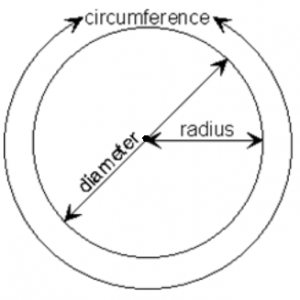The image above is a circle.

To compute the perimeter or circumference of a circle, one essential parameter is needed and this parameter is the radius of a circle (r). You can also use the diameter of a circle to compute the area of a circle (d).

The formula for calculating the perimeter or circumference of a circle is:

P = 2πr

Where:

P = Perimeter or Circumference of a circle
r = Radius of a circle

Let’s solve an example:
Find the perimeter or circumference of a circle where the radius of a circle is 8 cm.

This implies that;
r = Radius of a circle = 8 cm

P = 2πr
P = 2 x 3.142 x 8
P = 50.265

Therefore, the perimeter or circumference of a circle is 50.265 cm.

Calculating the Area of a Circle using the Diameter of a Circle.

The formula is P = πd

Where:

P = Perimeter or Circumference of a circle
d = Diameter of a circle

Let’s solve an example:
Find the perimeter or circumference of a circle where the diameter of a circle is 10 cm.

This implies that;
d = Diameter of a circle = 10 cm

P = πd
P = 3.142 x 10
P = 31.42

Therefore, the perimeter or circumference of a circle with diameter is 31.42 cm.

How to Calculate Radius of a Circle when Perimeter or Circumference of the Circle is Given

r = P /

where;

r = Radius of a circle
P = Perimeter or Circumference of a circle

Let’s solve an example:
Find the radius of a circle where the perimeter or circumference of the circle is 16 cm.

This implies that;
P = Perimeter or Circumference of the circle = 16 cm

r = P /
r = 16 / 6.284
r = 2.55

Therefore, the radius of the circle is 2.55 cm.

How to Calculate Diameter of a Circle when Perimeter or Circumference of the Circle is Given

d = P / π

where;

d = Diameter of a circle
P = Perimeter or Circumference of a circle

Let’s solve an example;
Find the diameter of a circle where the perimeter or circumference of the circle is 20 cm

This implies that;
P = Perimeter or Circumference of the circle = 20 cm

d = P / π
d = 20 / π
d = 6.365

Therefore, the diameter of the circle is 6.365 cm.

## How to Calculate and Solve for the Area and Length of a Parallelogram | The Calculator EncyclopediaThe image above represents a parallelogram.
To compute the area of a parallelogram requires two essential parameters which are the base and height of the parallelogram.

The formula for computing the area of a parallelogram is:

A = b x h

Where:
A = Area of a parallelogram
b = base of the parallelogram
h = height of the parallelogram

Let’s solve an example
Find the area of a parallelogram with a base of 12 cm and a height of 4 cm.

This implies that:
b = base of the parallelogram = 12
h = height of the parallelogram = 4

A = b x h
A = 12 x 4
A = 48

Therefore, the area of the parallelogram is 48 cm2.

Calculating the base of a parallelogram when Area and Height is Given

The formula is b = A / h

Where;
A = Area of a parallelogram
b = base of the parallelogram
h = height of the parallelogram

Let’s solve an example:
Find the base of a parallelogram with an area of 40 cm² and a height of 8 cm

This implies that;
A = Area of the parallelogram = 40 cm²
h = height of the parallelogram = 8 cm

b = A / h
b = 40 / 8
b = 5

Therefore, the base of the parallelogram is 5 cm.

Calculating the height of a parallelogram when Area and Base is Given

The formula is h = A / b

Where;
A = Area of a parallelogram
b = base of the parallelogram
h = height of the parallelogram

Let’s solve an example:
Find the height of a parallelogram with an area of 60 cm² and a base of 6 cm

This implies that;
A = Area of the parallelogram = 60 cm²
b = base of the parallelogram = 6 cm

h = A / b
h = 60 / 6
h = 10

Therefore, the height of the parallelogram is 10 cm.

## How to Calculate and Solve for the Area, Base and Height of a Triangle | The Calculator Encyclopedia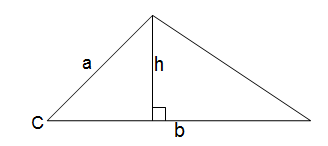The image above is a triangle.
To compute the area of a triangle two essential parameters are required which are the base of the triangle and the height of the triangle.

The formula for calculating the area of a triangle is:

A = 0.5bh

Where:

A = Area of a Triangle
b = Base of a Triangle
h = Height of a Triangle

Let’s solve an example:
Given that the base of a triangle is 12 cm and the height of the triangle is 4 cm. Find the area of the triangle.

This implies that:
b = Base of the Triangle = 12 cm
h = Height of the Triangle = 4 cm

A = 0.5bh
A = 0.5(12)(4)
A = 0.5(48)
A = 24

Therefore, the area of the triangle is 24 cm2

How to Calculate the Base of a Triangle when the Area and Height of the Triangle is Given

The formula is:

b = 2A / h

Where:

b = Base of the Triangle
A = Area of the Triangle
h = Height of the Triangle

Let’s take an example:
Find the base of a triangle when the height and area of the triangle is 12 cm and 40 cm2 respectively.

This implies that:
A = Area of the Triangle = 40 cm2
h = Height of the Triangle = 12 cm

b = 2(40) / 12
b = 80 / 12
b = 6.667

Therefore, the base of the triangle is 6.667 cm.

How to Calculate the Height of a Triangle when the Area and Base of the Triangle is Given

The formula is:

h = 2A / b

Where: